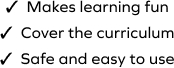# Subtraction Scenario

SplashLearn - The complete PreK - Grade 5 Math & ELA Learning Program Built for Your Child
Home > Subtraction Scenario
Boost your child's understanding of subtraction with this game. The game is about solving problems on subtraction by using real-world objects as visual help. In this game, students apply maths to real-world problems by working with a set of subtraction word problems. They work with "take away" scenarios. The game requires the students to make sense of each story situation and find the final answer.